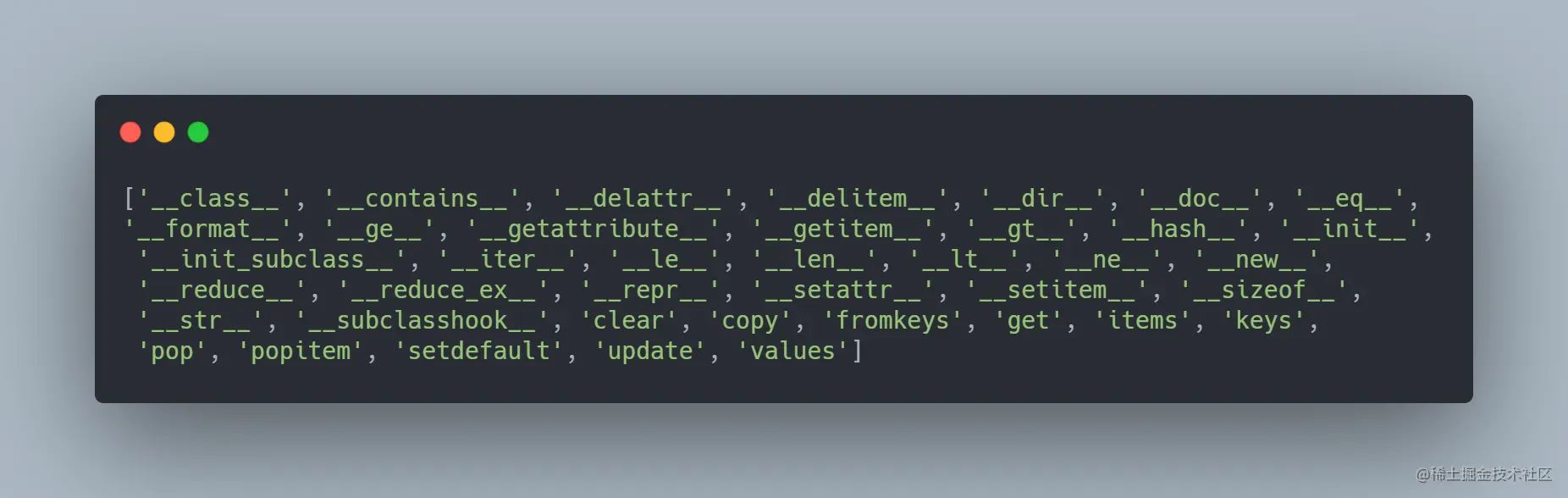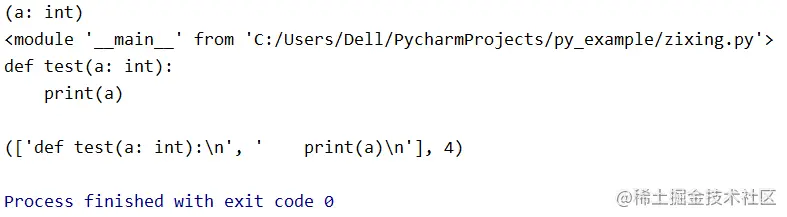# Python强大的自省机制详解

## dir()函数

```user = {"nickname": "tigeriaf", "level": 2}
print(dir(user))```## type()函数和id()函数

type()函数返回一个对象的类型。例如：

```print(type('tigeriaf'))
# 结果输出为 <class 'str'>
print(type(2))
# 结果输出为 <class 'int'>
print(type([1, 2, 3]))
# 结果输出为 <class 'list'>```

id()函数返回对象的唯一标识符，是一个整数，在CPython中id()函数用于获取对象的内存地址。例如：

```print(id('tigeriaf'))
```

# 结果输出为 51064768

## inspect模块

inspect是Python的标准库，提供了更加强大的自省能力，提供了很多函数帮助获取对象的信息，例如模块、类、方法、函数、回溯、帧对象以及代码对象。

### getmembers(object, predicate=None)函数

```import inspect
print(inspect.getmembers(list))```### getsourcelines(object)函数

```import inspect
def test(a: int):
print(a)
print(inspect.signature(test))
print(inspect.getmodule(test))
print(inspect.getsource(test))
print(inspect.getsourcelines(test))```## hasattr()函数和getattr()函数

dir()函数会返回某个对象所有属性的列表，但是如果只想测试一个或多个属性是否存在，就需要hasattr()函数和 getattr()函数来完成了，它们的功能分别为判断对象是否有某个属性、获得某个属性值。 例如：

```class MyObj:
def __init__(self):
self.name = 'my_obj'
self.a = 1
self.b = [1, 2, 3]
myobj = MyObj()
print(getattr(myobj, "name"))
print(getattr(myobj, "b"))
print(hasattr(myobj, "a"))
print(hasattr(myobj, "c"))```## 总结

0
0

0 条回复（回复会通过微信通知作者）

（微信扫码即可登录，无需注册）